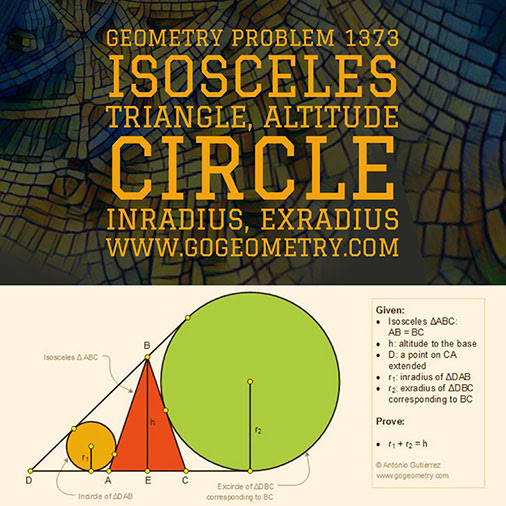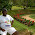## Saturday, August 4, 2018

### Typography of Geometry Problem 1373: Isosceles Triangle, Exterior Cevian, Inradius, Exradius, Altitude, iPad Apps

Geometric Art: Typography, iPad

Details: Click on the figure below.#### 1 comment:

1.Let the in circle be P and the excircle be Q
Let DAC touch circle P at X & circle Q at Y
Let DB touch circle P at U and circle Q at V

Let AB = BC = a, AX = b, CY = c and
AE = EC = d
Let the bisector of < BAE meet BE at T

ET = hd / (a + d) ......(1)

Now PXA, TEA & QYC are similar triangles
So b / r1 = c / r2 = ET / d = h / (a + d)
= (b + c) / ( r1 + r2) = (a - d) / (r1 + r2) from (1)

Hence (r1 + r2)h = a^2 - d^2 = h^2
Therefore r1 + r2 = h

Sumith Peiris
Moratuwa
Sri Lanka